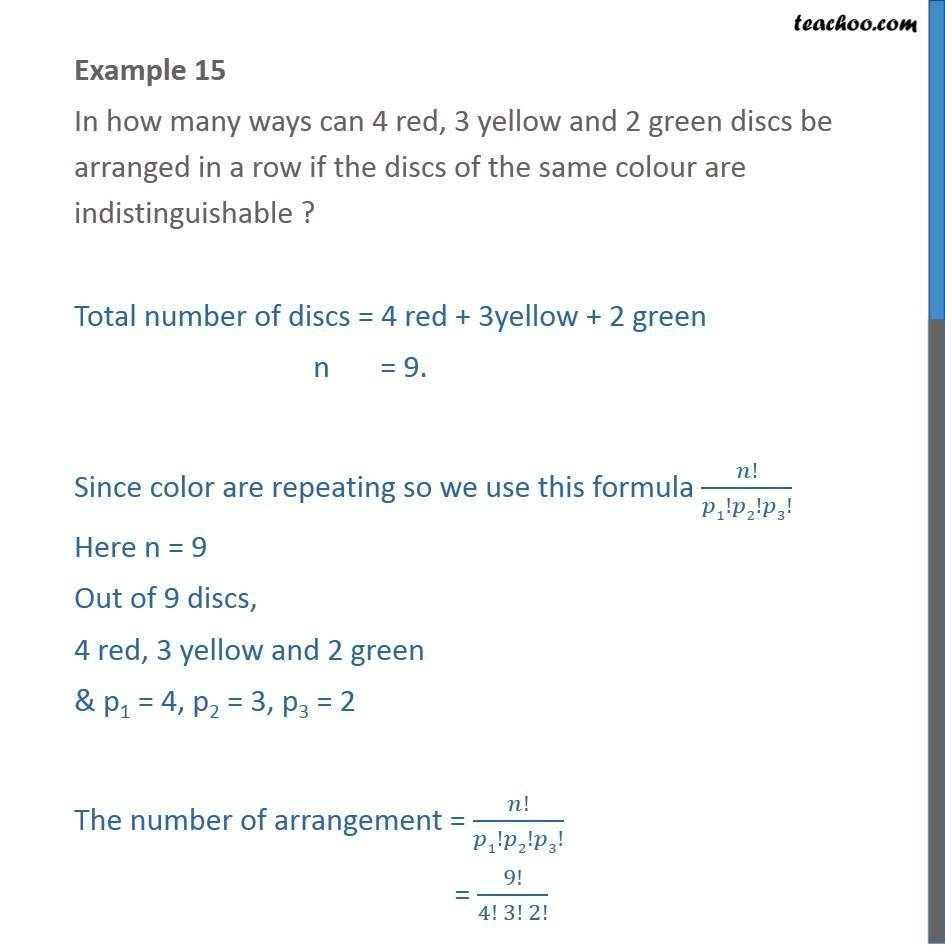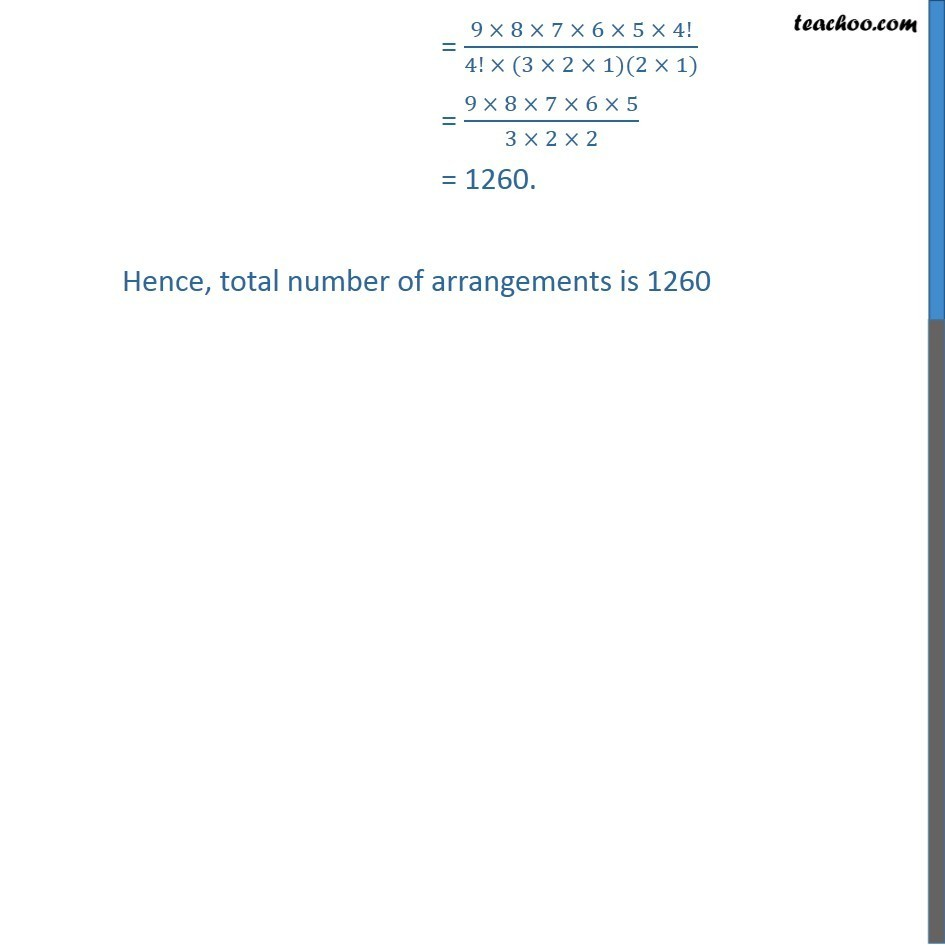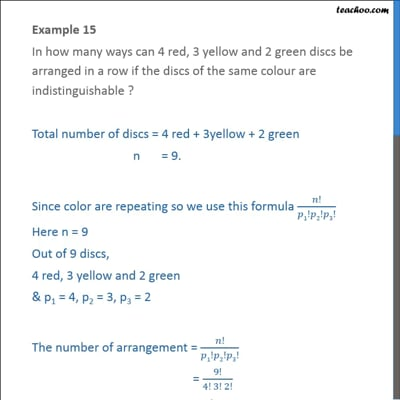Examples

Chapter 7 Class 11 Permutations and Combinations
Serial order wiseThis video is only available for Teachoo black users

Solve all your doubts with Teachoo Black (new monthly pack available now!)

### Transcript

Example 15 In how many ways can 4 red, 3 yellow and 2 green discs be arranged in a row if the discs of the same colour are indistinguishable ? Total number of discs = 4 red + 3yellow + 2 green n = 9. Since color are repeating so we use this formula 𝑛!/𝑝1!𝑝2!𝑝3! Here n = 9 Out of 9 discs, 4 red, 3 yellow and 2 green & p1 = 4, p2 = 3, p3 = 2 The number of arrangement = 𝑛!/𝑝1!𝑝2!𝑝3! = 9!/(4! 3! 2!) = (9 × 8 × 7 × 6 × 5 × 4!)/(4! × (3 × 2 × 1)(2 × 1)) = (9 × 8 × 7 × 6 × 5)/(3 × 2 × 2) = 1260. Hence, total number of arrangements is 1260